## The Human - Machine InterfaceAnd The Equilibrium Matrix

Please note that it might take a while to load some of the graphics on this page, and that it would be best to print this document in landscape mode in order to get all of the graphics on the page.

The circuit to be described here is the result of an effort to find electronics that mimic nature's tendency to seek equilibrium. The result is something called the Equilibrium Matrix, not to be confused with the equilibrium matrix sometimes used in other areas of study. Its most important potential use is in pattern recognition, especially in the effort to assist the disabled by means of human-machine interfaces. Everything presented in this document is provided free of charge to non-profits working to assist the disabled.

The Equilibrium Matrix consists entirely of analog components. All calculations required to transform input to output are performed in the analog domain. The only limitations are those imposed by the operational amplifiers used at each node. It is potentially capable of millions of transforms per second with no help from a microprocessor. Digital means are used only to obtain the results and to decide what to do with them.

Each node of the matrix consists of a differential amplifier which looks like the following in its basic form: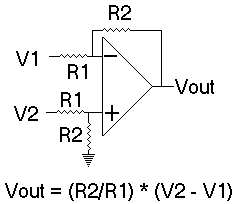The amplifier's output is simply the magnitude of the non-inverting input (V2) minus the magnitude of the inverting (V1) input if R1 = R2. Accuracy depends almost entirely on the precision of the resistors used. The most important factor for this application is the ratio of the resistors at each input. The two resistors at the inverting input must be as near the same as possible, and the two resistors at the non-inverting input must be as near the same as possible for the R1 = R2 case. There is no need however, for the resistors at the inverting input to be the same as those at the non-inverting input. The Equilibrium Matrix uses resistors contained in resistor network packages to take advantage of the close matching that comes from virtually the same time and same process of manufacture.

The resistor from the output to the inverting input is called the feedback resistor. The gain of the inverting input alone is:
-(Rfeedback / Rinput)
Since the input and feedback resistors are the same in the circuit above, the inverting gain is -1. Each node of the Equilibrium Matrix consists of a slightly modified version of this basic differential amplifier.

It uses input resistors that are 4 times larger than the feedback resistor, providing an inverting gain of -.25. There are four such inputs per node. The inverting side of the circuit uses a resistor network containing 9 resistors. One of the ends of all of the resistors are connected together internally and brought out to a single pin on a SIP (single in-line package)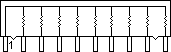such as the CTS Series 770. The free ends of the resistors are provided on 9 pins of the package. The common pin is connected to the inverting input of the amplifier. The free ends of four of the resistors are connected together and then to the output of the amplifier. Paralleling four resistors of the same value in this manner results in a combined resistance equal to 1/4th that of a single resistor. This parallel combination forms the feedback resistor. The free ends of four of the remaining resistors are used as inputs. The result provides a gain of -.25 for each of the inverting-side inputs. The four inputs are from adjacent nodes. They drive the output of the target node in a direction opposite to that of the output of an adjacent node, but at only 1/4th its level. Note that the ninth resistor is not normally used, although it could be used to apply an offset voltage to the nodes for systems that do not accept negative voltages.

Two of the eight resistors contained in a dual in-line package (DIP)such as the CTS Dual-In-Line Series 760/761 are used to construct the circuit at the non-inverting input, which remains unchanged from the basic circuit above: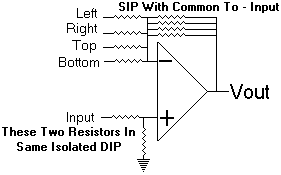The output of this circuit is:
Vout = Input - (Left + Right + Top + Bottom)/4

Consider the case where a node has a 1 volt input and is surrounded by four other nodes, each with 1 volt outputs. The output of the center node would be:
1 - (1 + 1+ 1+ 1)/4 = 0
The nodes in an Equilibrium Matrix are each driven in this manner. Adjacent nodes will tend to drive a central node in a direction opposite their values.

A node can be represented by a block diagram for simplification: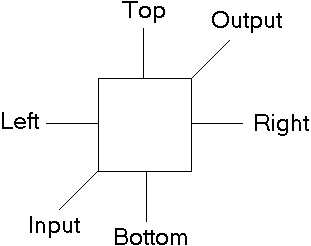The Equilibrium Matrix is an array of such nodes. The following block diagram represents a 3 X 3 matrix. A few interconnections are shown for clarity, but most are not for the same reason. Each node is designated by its row/column position, with node 1,1 being top-left. The left, right, top and bottom inputs to each node are designated by the coordinates of the source nodes. End nodes use the node at the opposite end of a row or column as an input to the side of the node facing the edge. Thus, node 1,1 uses node 1,3 as its left input and 3,1 as its top input: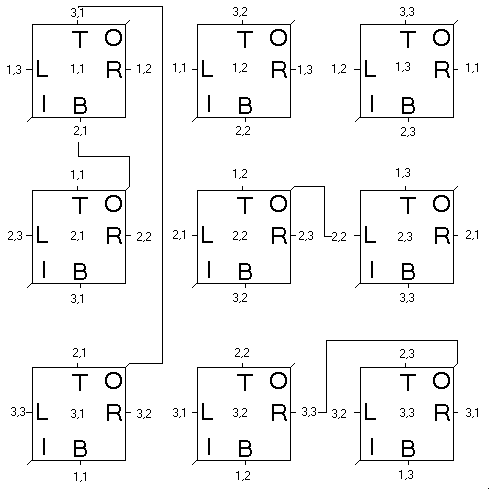With a fully interconnected matrix, the interleaving of the nodes causes all of them to be modified with a change of input to any, and the matrix will settle to a state of equilibrium when the inputs become stable. It is this combination that offers potential usefulness.

Outside-world voltages enter the non-inverting input of each node. The Equilibrium Matrix will respond by performing the summations necessary to find a state of equilibrium. Its outputs will represent the inputs plus the interaction of the nodes. The resulting information represents not only the node input values, but relational information among the nodes in a manner similar to a neural network with multiple feedback paths. The matrix can generate the information in a virtually real-time fashion with no need for external computations.

The result is a circuit that can perform much of the front-end work for pattern recognition. Oversimplified, the essence of pattern recognition is to take the values of points on a pattern, then determine the relationship among the values. The number of points considered determines the precision of recognition. The Equilibrium Matrix can yield a representation of the relationship among its input nodes, significantly reducing the work of a microprocessor.

The possibilities are endless. Many applications can be found in commercial pattern recognition systems. Since I can't afford a patent however, I will have to trust the integrity of those who seek commercial applications to provide appropriate remuneration. The most intriguing possibility however (and the main reason I am giving this information away to non-profits), is in non-profit efforts to assist the disabled.

Consider the situation where the Equilibrium Matrix is connected to the outputs of an EEG or EMG, the goal being to control a wheelchair. In the training mode, the person thinks of say, "turn right," or imagines the motor control required to cause the turn. The computer acquires several thousand digital conversions of the outputs of several nodes and stores them. The high and low values for each node monitored are determined and stored.

In the operational mode, the Equilibrium Matrix will again provide relational information among the nodes, and the computer will again obtain digital input from the same nodes used in training. There will however, be no need for the computer to perform intensive, time consuming calculations. It will simply look to see if the digital value of each monitored node falls inside the high and low training values for a right turn. If that is the case, output will be provided to turn the wheelchair. It might not prove to be that simple, but the process should provide a good starting point.

I have written some software that simulates an Equilibrium Matrix. A ziped file can be downloaded here. Just download it to a convenient directory on your PC and double click on it to extract the files. WinZip is used to unzip the file and can be downloaded here. Read the readme.txt file for instructions on using the program. The executible is for DOS, but source is also provided so it can be compiled for other machines and/or modified. It is common ANSI C code, so it should compile on virtually any machine.

Tests can easily be set up using various numbers of nodes as inputs to the simulator. Note that even numbers of both rows and columns cannot be used. Latchup and/or oscillation occurs when there are even numbers of both (try 4x4.txt as an input to see the effect).

A file with a series of matrices can be set up to simulate the results of multiple samples. The least and greatest value for each node is provided when a series is detected. They can be used to see what a training session might produce. A simulated operational matrix input can then be used to determine if there would have been a hit. See the series.txt file as an example.

The following is a pictorial to aid in the construction of nodes. The LF412 amplifier was used due to its low offset characteristics, which makes offset trimmers unnecessary ( see LF412.PDF ). It is a dual amplifier, so two nodes can be constructed with each package. V+ and V- can be up to +/- 15 volts. I used the +/- 12V from an old computer supply. I used 47K ohm units for the bussed resistor packs and 22K ohms for the isolated units. Almost any reasonable values could be used:Use the OS inputs to set an offset if needed. Tie all of them together and supply twice the desired offset. It should be possible to adjust the offset when no other inputs are connected.

One of the nice things about the Equilibrium Matrix is its low cost. The following is an example from one source. A 3X5 matrix is less than \$19.00 excluding the optional parts (at least it was when I last checked Jameco). A little shopping might produce even better prices. Another good source is Digi-Key. Search their site by part name or description.

 Quantity Part Jameco Number(highlight and paste in their searchthen add quantity to cart) Price for Quantity 10(2 extra for "oops, burned up" factor) LF412 23026 10.90 15 47K SIP Arrays 98087 2.85 8 10K DIP Arrays 108628 4.72 Subtotal for parts \$18.47 1 Optional breadboard 20811 31.95 1 Optional wire kit 19289 10.49 1 Optional refill for wire kit 20079 8.95 10 Optional Trimmers for testing 183564 27.50 Subtotal for optional items \$78.89 Total \$97.36

The following is a 3 X 3 Equilibrium Matrix that was constructed on a solderless breadboard. The trimmers near the bottom are 20-turn units that were used to provide test voltages to the nodes. Their clock-wise sides were connected to V+, and counter-clock-wise to V-:Data can be input to a computer with any of many analog to digital converters. The center node, the corner nodes and the nodes at the center of each edge might be reasonable places to start monitoring. That's all of the nodes on a 3x3 Equilibrium Matrix. The center node could be omitted for systems that have only 8 inputs -- a common number for many.

The following is an alphabetical listing of some places to look for analog to digital converters:
Analog Devices
Maxim
National Semiconductor

There are also a lot of ready-made analog to digital converter boards on the market. The folowing google search produced some promising results: analog to digital converter acquisition boards .

A couple of my sites are listed below, just in case anyone is interested. Note that the board offered at the tutorial site has an 8 input, 8-bit digital to analog converter (as well as analog output and digital I/O). It might work for testing, but I think higher resolution will be needed for actual use of the Equilibrium Matrix:
Control And Embedded Systems
Free Hands-on Tutorial Using C And An Inexpensive ISA Board
The Super Start Project
Giving Kids The Basic Core Knowledge They Need For A Good Beginning In Education